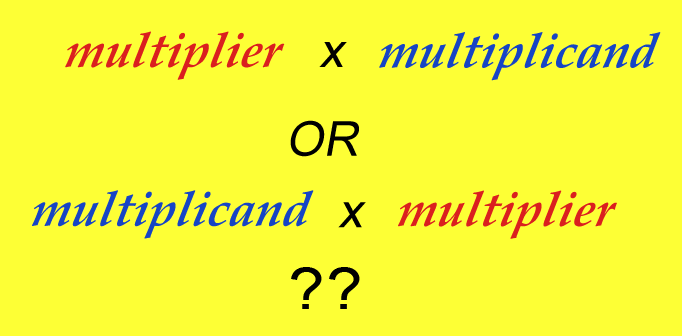^
You are here: HomeLessons → Multiplier & multiplicand

# Does the order of multiplicand and multiplier matter in a multiplication equation?

It seems that in Math Mammoth Multiplication 1 book, the multiplicand is second number and not the first. I had been taught that the equations are multiplicand x multiplier = product. I know that either order will produce the same product but is there a reason that you have the the equations multiplier x multiplicand? Just want to make sure that I am consistent when teaching my son.

In case you don't know, "multiplicand" is the number or quantity we are multiplying, and "multiplier" is the number we multiply by.Yes, I do have a reason for it. It has to do with what happens down the road a bit.

In 4th grade, students encounter the thought of a fractional part of a quantity, such as finding 3/4 of \$36. While we first use a two-step operation to find the answer, eventually (in 5th grade) students will learn how this corresponds to the fraction multiplication ¾ × 36.

Notice how the word "OF" from "3/4 of \$36" translates into MULTIPLICATION, and the multiplicand is written SECOND.

Also in 5th grade, students will learn about multiplication as a scaling operation. For example, if something is 50 cm long and it is scaled by the factor 1.2, the new length will be 1.2 × 50 cm = 60 cm.

This then ties in with PERCENTAGES. Scaling some quantity by 1.2 is the same as 120% of the quantity. As you know, 120% is the same as the decimal 1.2. We can then equate the word "of" in statements of "percentage of something" with multiplication operation:

```68% of \$450
↓   ↓   ↓
0.68 × \$450
```

This is extremely handy, not only with percents, but in algebra. Using this principle it is so easy to build algebraic equations such as:

0.9p = 5.6

where p is an unknown price so that when it's discounted by 10%, the new price is \$5.60.

Back to 3rd grade multiplication.... I want children to see multiplication in that order (multiplier × multiplicand) so that when they get to percentages, and encounter 68% of \$450, they will be able to quickly "translate" that to 0.68 × \$450 (where we also have the multiplicand as the 2nd number).

But, children don't need to learn the terms "multiplier" and "multiplicand". I am afraid they may just confuse the issue. It's sufficient to simply use the term "FACTOR" for both numbers.

By Maria Miller

WAIT!

Receive my monthly collection of math tips & resources directly in your inbox — and get a FREE Math Mammoth book!You can unsubscribe at any time.

### Math Mammoth Tour

Confused about the different options? Take a virtual email tour around Math Mammoth! You'll receive:

An initial email to download your GIFT of over 400 free worksheets and sample pages from my books. Six other "TOURSTOP" emails that explain the important things and commonly asked questions concerning Math Mammoth curriculum. (Find out the differences between all these different-colored series!)

This way, you'll have time to digest the information over one or two weeks, plus an opportunity to ask me personally about the curriculum.
A monthly collection of math teaching tips & Math Mammoth updates (unsubscribe any time)### "Mini" Math Teaching Course

This is a little "virtual" 2-week course, where you will receive emails on important topics on teaching math, including:

- How to help a student who is behind
- Troubles with word problems
- Teaching multiplication tables
- Why fractions are so difficult
- The value of mistakes
- Should you use timed tests
- And more!

A GIFT of over 400 free worksheets and sample pages from my books right in the very beginning.A monthly collection of math teaching tips & Math Mammoth updates (unsubscribe any time)
Enter your email to receive math teaching tips, resources, Math Mammoth news & sales, humor, and more! I tend to send out these tips about once monthly, near the beginning of the month, but occasionally you may hear from me twice per month (and sometimes less often).• A GIFT of over 400 free worksheets and sample pages from my books.